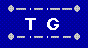Interesting Math Problem Math Problems Home  |  Home  |  Send Feedback

## Shared Work Calculator

This calculator will solve "shared work" problems,
which typically go like this:

Alice can do the work (paint a room, type some letters, etc.) in 2 hours.
If Bob helps, they can finish the job in 75 minutes.
How long would it take Bob to do it on his own?

The solution is along these lines:

Alice does 1/120 of the job in 1 minute.
Together they do 1/75 of the job in 1 minute.
So in 1 minute, Bob does 1/75 - 1/120 = 1/200 of the job.
So Bob takes "1 over that", or 200 minutes to do the job.

If we are given the time it takes each worker,
and want the time taken working together,
we use the equation 1/worker1 + 1/worker2 = 1/together,
and "1 over that" equals time taken together.
For the above problem: 1/120 + 1/200 = 1/75.

Here is another approach, based on fraction of the work done:

Let the rate of worker1 be r, and the rate of worker2 be s.
When they work together, let w = fraction of the work done by worker1.
Then we have w + w * (r/s) = 1 and w = 1 / (1 + r/s).

In the above example, where Alice takes 120 minutes and Bob takes 200,
for Alice we have, w = 1 / (1 + 120/200) = 1 / (320/200) = 5/8.
for Bob we have, w = 1 / (1 + 200/120) = 1 / (320/120) = 3/8.
So when they are working together, Alice does 5/8 of the work,
and Bob does 3/8 of the work, and it takes
5/8 * 120 = 3/8 * 200 = 75 minutes to complete the job.

Enter numbers into any 2 of the 3 boxes
then click "solve" to determine the third one.

 Time units required for worker 1: Time units required for worker 2: Time units if they work together: